Documentation

## Multisignal 1-D Wavelet Analysis

A 1-D multisignal is a set of 1-D signals of same length stored as a matrix organized rowwise (or columnwise).

The purpose of this example is to show how to analyze, denoise or compress a multisignal, and then to cluster different representations or simplified versions of the signals composing the multisignal.

In this example, the signals are first analyzed and different representations or simplified versions are produced:

• Reconstructed approximations at given levels,

• Denoised versions,

• Compressed versions.

Denoising and compressing are two of the main applications of wavelets, often used as a preprocessing step before clustering.

The last step of this example performs several clustering strategies and compare them. It allows to summarize a large set of signals using sparse wavelet representations.

### Load and Plot a Multisignal

To illustrate these capabilities, let us consider a real-life multisignal representing 35 days of an electrical load consumption, centered and standardized.

```load elec35_nor; % Load normalized original data. X = signals; [nbSIG,nbVAL] = size(X); plot(X','r'); axis tight title('Original Data: 35 days of electrical consumption'); xlabel('Minutes from 1 to 1440'); ```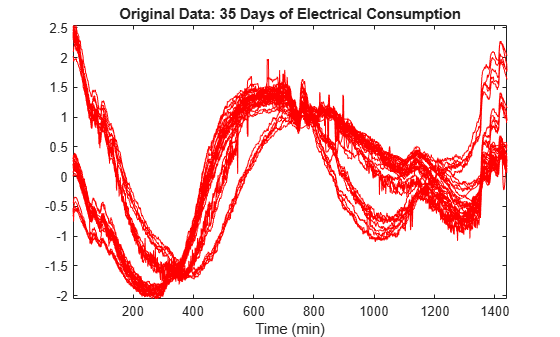We can see that the signals are locally irregular and noisy but nevertheless three different general shapes can be distinguished.

### Surface Representation of Data

In order to highlight the periodicity of the multisignal, let us now examine the data using a 3-D representation.

```surf(X); shading interp axis tight title('Original Data: 35 days of electrical consumption'); xlabel('Minutes from 1 to 1440','Rotation',4); ylabel('Days from 1 to 35','Rotation',-60); ax = gca; ax.View = [-13.5 48]; ```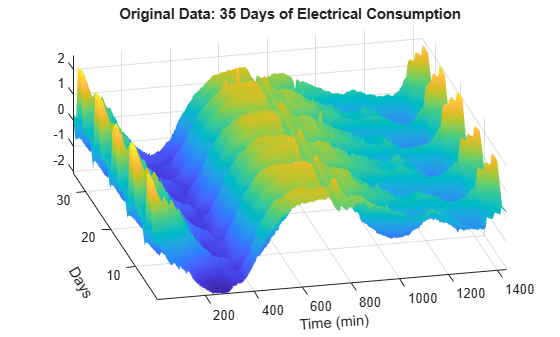The five weeks represented can now be seen more clearly.

### Multisignal Row Decomposition

Perform a wavelet decomposition at level 7 using the ' sym4 ' wavelet.

```dirDec = 'r'; % Direction of decomposition level = 7; % Level of decomposition wname = 'sym4'; % Near symmetric wavelet decROW = mdwtdec(dirDec,X,level,wname); ```

The generated decomposition structure is as follows:

```decROW ```
```decROW = struct with fields: dirDec: 'r' level: 7 wname: 'sym4' dwtFilters: [1x1 struct] dwtEXTM: 'sym' dwtShift: 0 dataSize: [35 1440] ca: [35x18 double] cd: {1x7 cell} ```

### Signals and Approximations at Level 7

Let us reconstruct the approximations at level 7 for each row signal. Then, to compare the approximations with the original signals, let us display two plots (see figure below). The first one shows all the original signals and the second one shows all the corresponding approximations at level 7.

```A7_ROW = mdwtrec(decROW,'a',7); subplot(2,1,1); plot(X(:,:)','r'); title('Original Data'); axis tight subplot(2,1,2); plot(A7_ROW(:,:)','b'); axis tight title('Corresponding approximations at level 7'); ```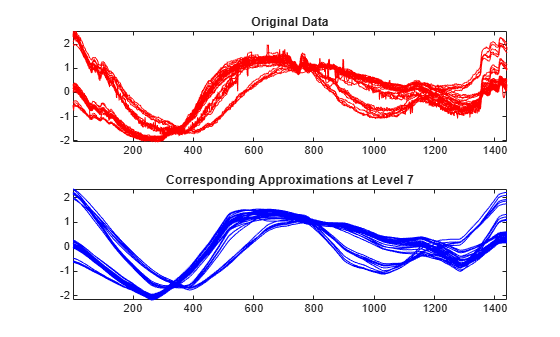As it can be seen, the general shape is captured by the approximations at level 7, but some interesting features are lost. For example, the bumps at the beginning and at the end of the signals disappeared.

### Superimposed Signals and Approximations

To compare more closely the original signals with their corresponding approximations at level 7, let us display two plots (see figure below). The first one concerns the four first signals and the second one the five last signals. Each of these plots represents the original signals superimposed with their corresponding approximation at level 7.

```subplot(2,1,1); idxDays = 1:4; plot(X(idxDays,:)','r'); hold on plot(A7_ROW(idxDays,:)','b'); axis tight title(['Days ' int2str(idxDays), ' - Signals and Approximations at level 7']); subplot(2,1,2); idxDays = 31:35; plot(X(idxDays,:)','r'); hold on plot(A7_ROW(idxDays,:)','b'); axis tight title(['Days ' int2str(idxDays), ' - Signals and Approximations at level 7']); ```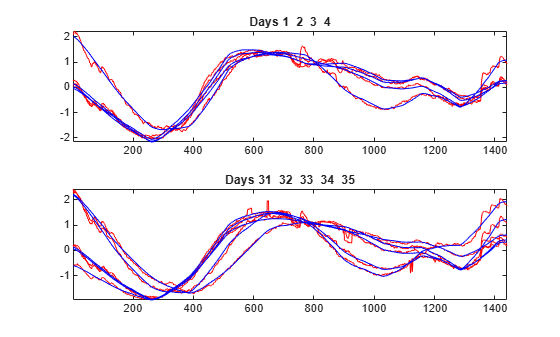As it can be seen, the approximation of the original signal is visually accurate in terms of general shape.

### Multisignal Denoising

To perform a more subtle simplification of the multisignal preserving these bumps which are clearly attributable to the electrical signal, let us denoise the multisignal.

The denoising procedure is performed using three steps:

1) Decomposition : Choose a wavelet and a level of decomposition N, and then compute the wavelet decompositions of the signals at level N.

2) Thresholding : For each level from 1 to N and for each signal, a threshold is selected and thresholding is applied to the detail coefficients.

3) Reconstruction : Compute wavelet reconstructions using the original approximation coefficients of level N and the modified detail coefficients of levels from 1 to N.

Let us choose now the level of decomposition N = 5 instead of N = 7 used previously.

```dirDec = 'r'; % Direction of decomposition level = 5; % Level of decomposition wname = 'sym4'; % Near symmetric wavelet decROW = mdwtdec(dirDec,X,level,wname); [XD,decDEN] = mswden('den',decROW,'sqtwolog','mln'); Residuals = X-XD; subplot(3,1,1); plot(X','r'); axis tight title('Original Data: 35 days of electrical consumption'); subplot(3,1,2); plot(XD','b'); axis tight title('Denoised Data: 35 days of electrical consumption'); subplot(3,1,3); plot(Residuals','k'); axis tight title('Residuals'); xlabel('Minutes from 1 to 1440'); ```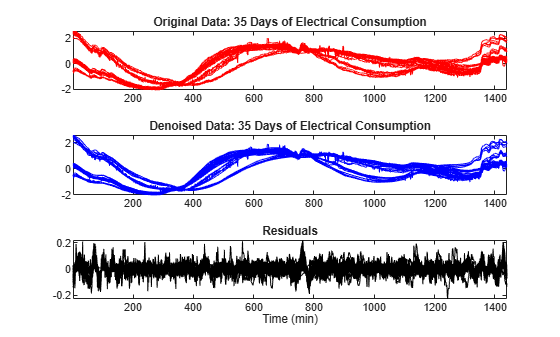The quality of the results is good. The bumps at the beginning and at the end of the signals are well recovered and, conversely, the residuals look like a noise except for some remaining bumps due to the signals. Furthermore, the magnitude of these remaining bumps is of a small order.

### Multisignal Compressing Row Signals

Like denoising, the compression procedure is performed using three steps (see above).

The difference with the denoising procedure is found in step 2. There are two compression approaches available:

• The first one consists of taking the wavelet expansions of the signals and keeping the largest absolute value coefficients. In this case, you can set a global threshold, a compression performance, or a relative square norm recovery performance. Thus, only a single signal-dependent parameter needs to be selected.

• The second approach consists of applying visually determined level-dependent thresholds.

To simplify the data representation and to make more efficient the compression, let us go back to the decomposition at level 7 and compress each row of the multisignal by applying a global threshold leading to recover 99% of the energy.

```dirDec = 'r'; % Direction of decomposition level = 7; % Level of decomposition wname = 'sym4'; % Near symmetric wavelet decROW = mdwtdec(dirDec,X,level,wname); [XC,decCMP,THRESH] = mswcmp('cmp',decROW,'L2_perf',99); subplot(3,1,1); plot(X','r'); axis tight title('Original Data: 35 days of electrical consumption'); subplot(3,1,2); plot(XC','b'); axis tight title('Compressed Data: 35 days of electrical consumption'); subplot(3,1,3); plot((X-XC)','k'); axis tight title('Residuals'); xlabel('Minutes from 1 to 1440'); ```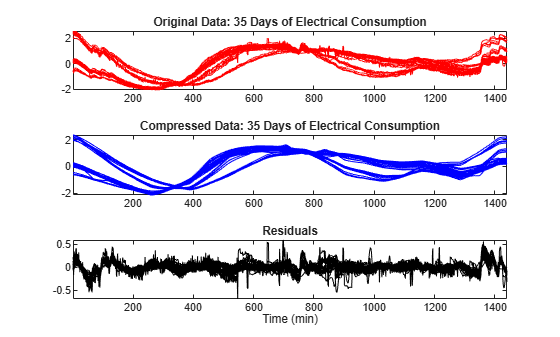As it can be seen, the general shape is preserved while the local irregularities are neglected. The residuals contain noise as well as components due to the small scales essentially.

### Compression Performance

Let us now compute the corresponding densities of nonzero elements.

```cfs = cat(2,[decCMP.cd{:},decCMP.ca]); cfs = sparse(cfs); perf = zeros(1,nbSIG); for k = 1:nbSIG perf(k) = 100*nnz(cfs(k,:))/nbVAL; end figure('Color','w') plot(perf,'r-*'); title('Percentages of nonzero coefficients for the 35 days'); xlabel('Signal indices'); ylabel('% of nonzero coefficients'); ```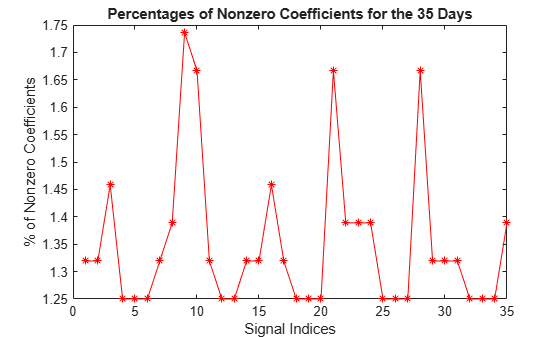For each signal, the percentage of required coefficients to recover 99% of the energy lies between 1.25% and 1.75%. This illustrates the capacity of wavelets to concentrate signal energy in few coefficients.

### Clustering Row Signals

Clustering offers a convenient procedure to summarize a large set of signals using sparse wavelet representations. You can implement hierarchical clustering using `mdwtcluster`. You must have the Statistics and Machine Learning Toolbox™ to use `mdwtcluster`.

Compare three different clusterings of the 35-day data. The first one is based on the original multisignal, the second one on the approximation coefficients at level 7, and the last one is based on the denoised multisignal.

Set to the number of clusters to 3. Compute the structures P1 and P2 which contain respectively the two first partitions and the third one.

```P1 = mdwtcluster(decROW,'lst2clu',{'s','ca7'},'maxclust',3); P2 = mdwtcluster(decDEN,'lst2clu',{'s'},'maxclust',3); Clusters = [P1.IdxCLU P2.IdxCLU]; ```

We can now test the equality of the three partitions.

```EqualPART = isequal(max(diff(Clusters,[],2)),[0 0]) ```
```EqualPART = logical 1 ```

So the three partitions are the same. Let us now plot and examine the clusters.

```figure('Color','w') stem(Clusters,'filled','b:') title('The three clusters of the original 35 days'); xlabel('Signal indices'); ylabel('Index of cluster'); ax = gca; xlim([1 35]); ylim([0.5 3.1]); ax.YTick = 1:3; ```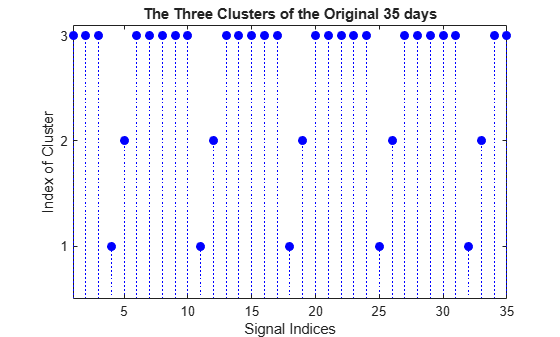The first cluster (labelled 3) contains 25 mid-week days and the two others (labelled 2 and 1) contain five Saturdays and five Sundays respectively. This illustrates again the periodicity of the underlying time series and the three different general shapes seen in the two first plots displayed at the beginning of this example.

Let us now display the original signals and the corresponding approximation coefficients used to obtain two of the three partitions.

```CA7 = mdwtrec(decROW,'ca'); IdxInCluster = cell(1,3); for k = 1:3 IdxInCluster{k} = find(P2.IdxCLU==k); end figure('Color','w','Units','normalized','Position',[0.2 0.2 0.6 0.6]) for k = 1:3 idxK = IdxInCluster{k}; subplot(2,3,k); plot(X(idxK,:)','r'); axis tight ax = gca; ax.XTick = [200 800 1400]; if k==2 title('Original signals'); end xlabel(['Cluster: ' int2str(k) ' (' int2str(length(idxK)) ')']); subplot(2,3,k+3); plot(CA7(idxK,:)','b'); axis tight if k==2 title('Coefficients of approximations at level 7'); end xlabel(['Cluster: ' int2str(k) ' (' int2str(length(idxK)) ')']); end ```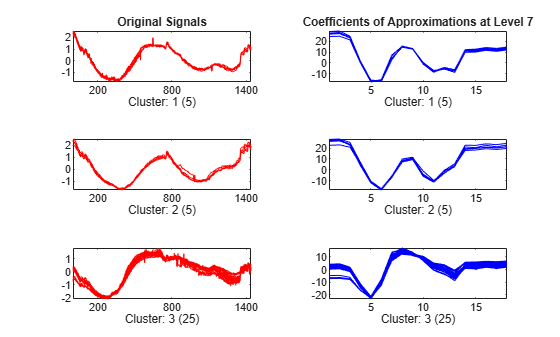The same partitions are obtained from the original signals (1440 samples for each signal) and from the coefficients of approximations at level 7 (18 samples for each signal). This illustrates that using less than 2% of the coefficients is enough to get the same clustering partitions of the 35 days.

### Summary

Denoising, compression and clustering using wavelets are very efficient tools. The capacity of wavelet representations to concentrate signal energy in few coefficients is the key of efficiency. In addition, clustering offers a convenient procedure to summarize a large set of signals using sparse wavelet representations.

##### Support평가판 신청